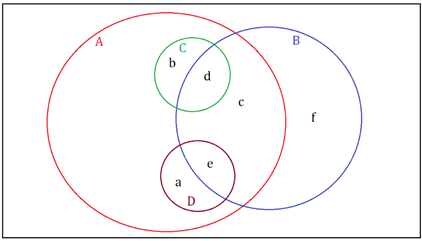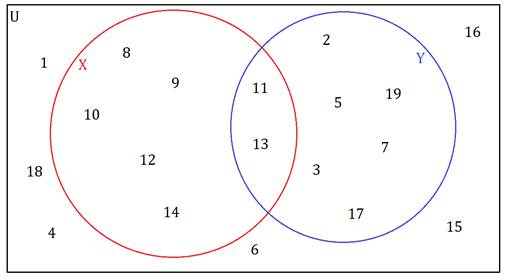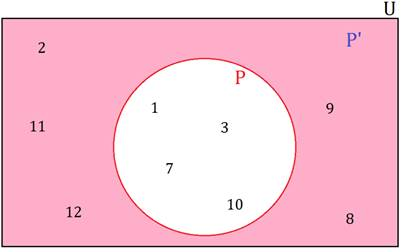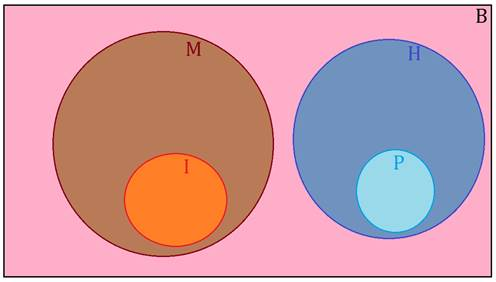SSC BOARD PAPERS IMPORTANT TOPICS COVERED FOR BOARD EXAM 2024

### Practice set 1.3 Sets Class 9th Mathematics Part I MHB Solution

###### Practice Set 1.3

Question 1.

If A = {a, b, c, d, e}, B = {c, d, e, f}, C = {b, d} and D = {a, e}.

then which of the following statements are true and which are false?

i. C ⊆ B ii. A ⊆ D
iii. D ⊆ B iv. D ⊆ A

v. B ⊆ A vi. C ⊆ A

We consider the following Venn diagram to check whether the above statements are true or not.Now, we have A = {a, b, c, d, e}, B = {c, d, e, f}, C = {b, d} and D = {a, e}.

So, it is clear from above that every element of set C and set D is also an element of set A.

∴ C ⊆ A and D ⊆ A

Hence, (iv), (vi) are true and (ii) is false.

Also, it is clear from above that every element of set B is not an element of set A.

∴ (v) is false.

Similarly, it is clear that every element of set C is not an element of set B.

∴ (i) is false.

Also, every element of set D is not an element of set B.

∴ (iii) is false.

Question 2.

Take the set of natural numbers from 1 to 20 as universal set and show set X and Y using Venn diagram.

i. X = {x | x ∈ ℕ, and 7 < x < 15}

ii. Y = {y | y ∈ ℕ, y is prime number from 1 to 20}

Consider the universal set:

U = {u | u ∈ ℕ, 1 ≤ x ≤ 20}

= {1, 2, 3, 4, 5, 6, 7, 8, 9, 10, 11, 12, 13, 14, 15, 16, 17, 18, 19, 20}

X = {x | x ∈ ℕ, 7 < x < 15}

= {8, 9, 10, 11, 12, 13, 14}

Y = {y | y ∈ ℕ, y is prime number from 1 to 20}

= {2, 3, 5, 7, 11, 13, 17, 19}Question 3.

U = {1, 2, 3, 7, 8, 9, 10, 11, 12}

P = {1, 3, 7, 10}

then (i) show the sets U, P and P � by Venn diagram. (ii) Verify (P’)’ = P

U = {1, 2, 3, 7, 8, 9, 10, 11, 12}

P = {1, 3, 7, 10}

P’ = {x | x ∈ U and x ∉ P} = {2, 8, 9, 11, 12}

(i) The Venn diagram showing U, P and P’ is:(ii) Now, we consider (P’)’

(P’)’ = {x | x ∈ U and x ∉ P’}

= {1, 3, 7, 10}

= P

Hence, (P’)’ = P.

Question 4.

A = {1, 3, 2, 7} then write any three subsets of A.

We know that any set S such that every element of S is an element of the set A is said to be a subset of A.

∴ Consider B = {1, 2, 3}, C = {1, 3} and D = {2, 3, 7}

It is clear from above that every element of the sets B, C and D is an element of set A, so, B, C and D are subsets of A.

Also, note that

Every set is a subset of itself, i. e. A ⊆ A and

Empty set is a subset of every set, i. e. ϕ ⊆ A.

So, A and ϕ are also subsets of A.

Question 5.

(i) Write the subset relation between the sets.

P is the set of all residents in Pune.

I is the set of all residents in Indore.

B is the set of all residents in India.

H is the set of all residents in Maharashtra.

(ii) Which set can be the universal set for above sets?

(i) Consider the following Venn diagram for the above sets:(ii) From the above Venn diagram, it is clear that set B can serve as the universal set for above sets.

Question 6.

Which set of numbers could be the universal set for the sets given below?

i. A = set of multiples of 5,

B = set of multiples of 7.

C = set of multiples of 12

A universal set is the set which can accommodate all other sets in it, i.e. every element of all the sets should be an element of the universal set.

A = set of multiples of 5,

B = set of multiples of 7.

C = set of multiples of 12

Consider the set of all integers, I = {………, -2, -1, 0, 1, 2,………}

We see that every element of the sets A, B and C is an element of the set I.

So, set I can serve as the universal set for the sets A, B and C.

Question 7.

P = set of integers which multiples of are 4.

T = set of all even square numbers.

A universal set is the set which can accommodate all other sets in it, i.e. every element of all the sets should be an element of the universal set.

P = set of integers which are multiples of 4

= {x | x ∈ ℤ and x is a multiple of 4}

T = {y | y is an even square number}

Consider the set of all integers, I = {………, -2, -1, 0, 1, 2,………}

We see that every element of both the sets P and T is an element of the set I.

So, set I can serve as the universal set for the sets P and T.

Question 8.

Let all the students of a class is a Universal set. Let set A be the students who secure 50% or more marks in Maths. Then write the complement of set A.

Let U be the universal set.

Then, U = Set of all students of a class

A = Set of students who secure 50% or more marks in Maths

We know that a set A’ is said to be the complement of the set A if it contains all the elements of the universal set U, which are not in the set A.

∴The complement of set A is:

A’ = Set of all students who do not secure 50% or more marks in Maths

Or A’ = Set of all students who secured less than 50% marks in Maths
∴ A'= U - A